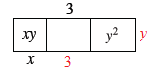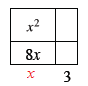### Home > INT1 > Chapter 4 > Lesson 4.1.3 > Problem4-27

4-27.

For each area model, fill in the missing parts. Write an equation showing that the area as a sum equals the area as a product.

1.  generic rectangle top edge middle $+3$ interior left$xy$ interior middle blank interterior left$y^2$ bottom edge left$x$
• The product of the dimensions of the rectangle is equal to the area.

•• $y(x + 3 + y) = xy + 3y + y^2$

1.  generic rectangle interior right top$x^2$ interior left top blank interior left bottom$8x$ interior right bottom blank bottom edge left blank bottom edge right$+3$

Follow the same step as (a).